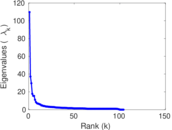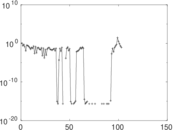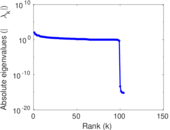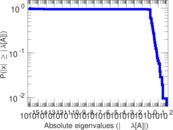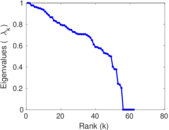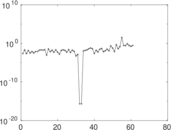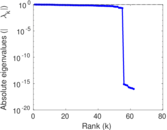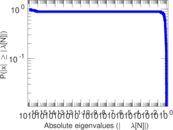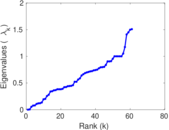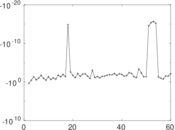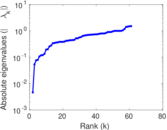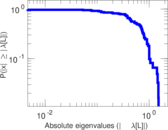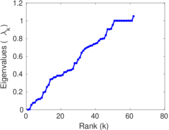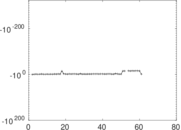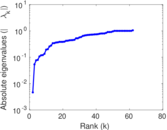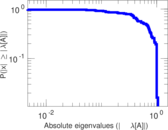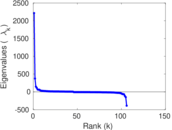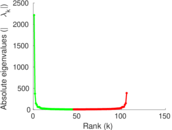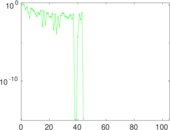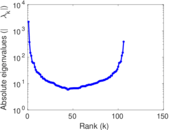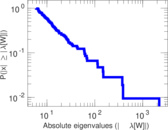# Wikinews edits (sd)

This is the bipartite edit network of the Sindhi Wikinews. It contains users and pages from the Sindhi Wikinews, connected by edit events. Each edge represents an edit. The dataset includes the timestamp of each edit.

 Code `nsd` Internal name `edit-sdwikinews` Name Wikinews edits (sd) Data source http://dumps.wikimedia.org/ AvailabilityDataset is available for download Consistency checkDataset passed all tests Category Authorship network Dataset timestamp 2017-10-20 Node meaning User, article Edge meaning Edit Network formatBipartite, undirected Edge typeUnweighted, multiple edges Temporal dataEdges are annotated with timestamps

## Statistics

 Size n = 2,208 Left size n1 = 106 Right size n2 = 2,102 Volume m = 4,069 Unique edge count m̿ = 2,753 Wedge count s = 1,276,118 Claw count z = 600,985,303 Cross count x = 224,571,666,148 Square count q = 82,324 4-Tour count T4 = 5,776,318 Maximum degree dmax = 2,495 Maximum left degree d1max = 2,495 Maximum right degree d2max = 66 Average degree d = 3.685 69 Average left degree d1 = 38.386 8 Average right degree d2 = 1.935 78 Fill p = 0.012 355 7 Average edge multiplicity m̃ = 1.478 02 Size of LCC N = 2,011 Diameter δ = 15 50-Percentile effective diameter δ0.5 = 1.865 01 90-Percentile effective diameter δ0.9 = 5.450 67 Median distance δM = 2 Mean distance δm = 3.215 22 Gini coefficient G = 0.665 201 Balanced inequality ratio P = 0.251 905 Left balanced inequality ratio P1 = 0.084 050 1 Right balanced inequality ratio P2 = 0.374 539 Relative edge distribution entropy Her = 0.705 490 Power law exponent γ = 4.992 54 Tail power law exponent γt = 2.621 00 Tail power law exponent with p γ3 = 2.621 00 p-value p = 0.000 00 Left tail power law exponent with p γ3,1 = 1.681 00 Left p-value p1 = 0.293 000 Right tail power law exponent with p γ3,2 = 5.841 00 Right p-value p2 = 0.478 000 Degree assortativity ρ = −0.218 982 Degree assortativity p-value pρ = 3.041 95 × 10−31 Spectral norm α = 109.703 Algebraic connectivity a = 0.004 623 28 Spectral separation |λ1[A] / λ2[A]| = 2.943 52 Controllability C = 1,980 Relative controllability Cr = 0.909 091

## Plots

### Fruchterman–Reingold graph drawing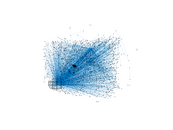### Degree distribution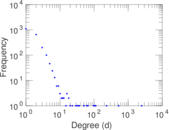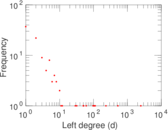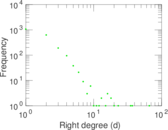### Cumulative degree distribution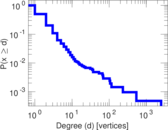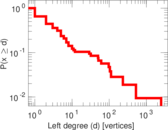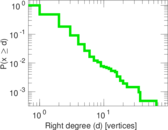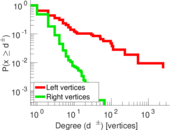### Lorenz curve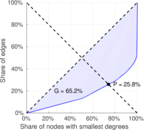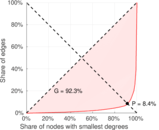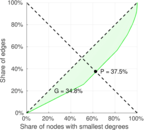### Spectral distribution of the adjacency matrix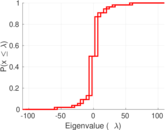### Spectral distribution of the normalized adjacency matrix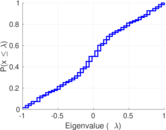### Spectral distribution of the Laplacian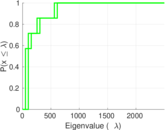### Spectral graph drawing based on the adjacency matrix### Spectral graph drawing based on the Laplacian### Spectral graph drawing based on the normalized adjacency matrix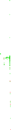### Degree assortativity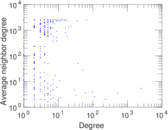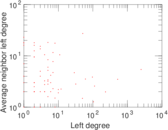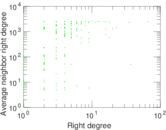### Zipf plot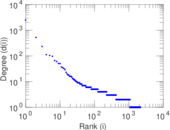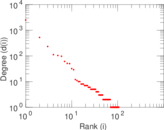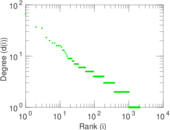### Hop distribution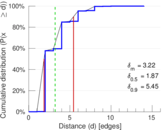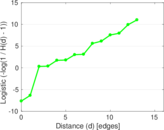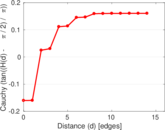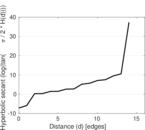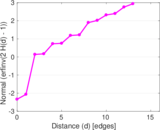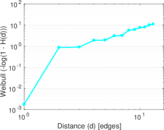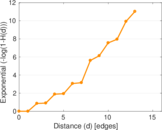### Double Laplacian graph drawing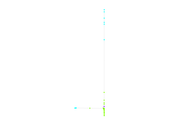### Delaunay graph drawing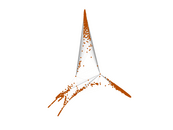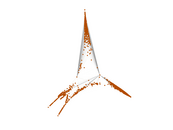### Edge weight/multiplicity distribution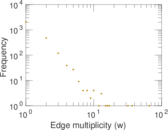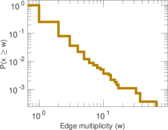### Temporal distribution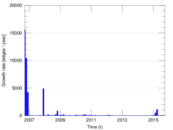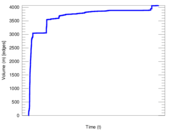### Temporal hop distribution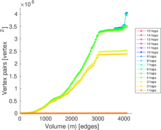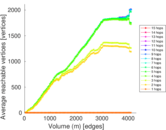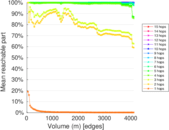### Diameter/density evolution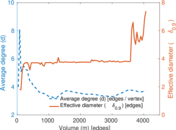### Matrix decompositions plots